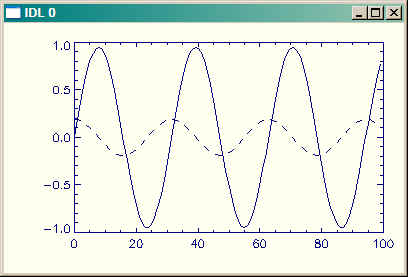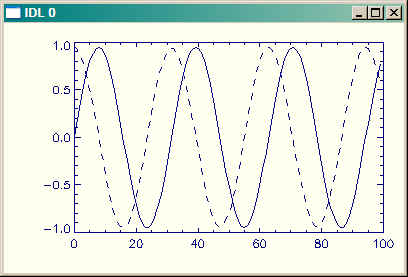Calculating Derivatives in IDL

QUESTION: I'm having trouble understanding how to calculate derivatives in IDL using the DERIV function? For example, suppose I create a data set like this:

data = Findgen(100)
y = 0.95 * Sin(data/5)

I know that the derivative of Sin(X) is Cos(X), so I would expect if I plot DERIV(Y) I should see a cosine function.

Plot, y
dx = DERIV(y)
OPlot, dx, LineStyle=2

In fact, I do, but the scale is vastly smaller than what I expect. How come?The derivative is correct, but seems to be on the wrong scale. Why?ANSWER: We know, by the chain rule that:

d/dx(f(g(x))) = f'(g(x))*g'(x)

d/dx(Sin(data/5)) = Cos(data/5) * (1/5)

The left-hand side of this equation is DERIV(Y), so you can see that if you simply multiply your answer by 5 that you will have what you want.

Plot, y
dx = DERIV(y)
OPlot, dx * 5, LineStyle=2Now the derivative is plotted at the correct scale.

Another way to find the correct answer would be to pass the function you are differentiating to the DERIV function, like this:

data = Findgen(100)
y = 0.95 * Sin(data/5)
Plot, y
dx = DERIV(data/5, y)
OPlot, dx, LineStyle=2

Or, even better, perform the division first, like this:

data = Findgen(100) / 5
y = 0.95 * Sin(data)
Plot, y
dx = DERIV(data, y)
OPlot, dx, LineStyle=2Web Coyote's Guide to IDL Programming